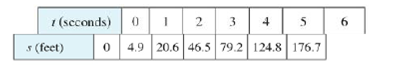Chapter 2.1, Problem 7E### Single Variable Calculus: Early Tr...

8th Edition
James Stewart
ISBN: 9781305270343

#### Solutions

Chapter
Section### Single Variable Calculus: Early Tr...

8th Edition
James Stewart
ISBN: 9781305270343
Textbook Problem

# The table shows the position of a motorcyclist after accelerating from rest.(a) Find the average velocity for each tune period:(i) [2, 4](ii) [3, 4](iii) [4, 5](iv) [4, 6](b) Use the graph of s as a function of t to estimate the instantaneous velocity when t = 3.

(a)

To determine

To find: The average velocity for given time periods.

Explanation

Formula used:

The average velocity over the time interval [t,t+h] is,

vavg=s(t+h)s(t)(t+h)t (1)

Calculation:

Section-(i)

Obtain the average velocity over the time interval [2, 4].

Substitute t=2 and t+h=4 in equation (1),

vavg=s(t+h)s(t)(t+h)t=s(4)s(2)42=s(4)s(2)2

From the given table, it is observed that,

(i) When t=2 seconds, the value of s(2)=20.6 ft.

(ii) When t=4 seconds, the value of s(4)=79.2 ft.

Thus, the average velocity over the time interval [2, 4] is computed as follows.

vavg=s(4)s(2)2=79.220.62=58.62=29.3

Therefore, the average velocity over the time interval [2, 4] is 29.3 ft/s.

Section-(ii)

Obtain the average velocity over the time interval [3, 4].

Substitute t=3 and t+h=4 in equation (1),

vavg=s(t+h)s(t)(t+h)t=s(4)s(3)43=s(4)s(3)1

From the given table, it is observed that,

(i) When t=3 seconds, the value of s(3)=46.5 ft.

(ii) When t=4 seconds, the value of s(4)=79.2.

Thus, the average velocity over the time interval [3, 4] is computed as follows.

vavg=s(4)s(3)=79

(b)

To determine

To estimate: The instantaneous velocity when t=3.

### Still sussing out bartleby?

Check out a sample textbook solution.

See a sample solution

#### The Solution to Your Study Problems

Bartleby provides explanations to thousands of textbook problems written by our experts, many with advanced degrees!

Get Started

#### Find more solutions based on key concepts